# Stacks of Maths!

##### Age 5 to 14

Published September 2007,February 2011.

I'm going to be looking at a piece of Maths that's quite simple and then see how this can be extended for much more experienced pupils.

(In this article, when I present the start of a number pattern and various activities that might be followed up, I show the first few parts only, as an example. More experienced pupils will be able to take it further by working at the numbers involved or by making use of a spreadsheet. If a fuller version would be helpful to you - maybe because the pupils would be good at and/or enjoy the number exploration but unable to extend it themselves - you can find this in the Notes section.)

It's all about stacking. So, for the youngest of our pupils we may be think about stacking cans in this way, I've called it "simple";
A.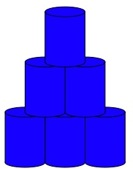So there are all the explorations about the number of cans needed for a triangle like this, the numbers for each new layer and the total number of cans needed for each bigger size of triangle. This will probably be recognised by you as the triangular numbers.

B. Then there's stacking boxes;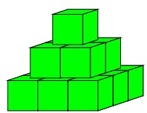So now we have each layer as a square number, and for some pupils it would be a good way to introduce the whole idea of square numbers. Again this can be explored by looking at layers and totals for each bigger size of square-based pyramid.

C. Then there's stacking balls so that a ball sits on three that are below it!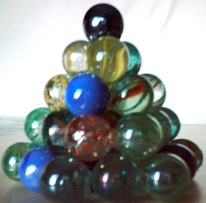(Had to be a photo - too hard to draw properly!)

Looking from above you get something like this for the first seven layers: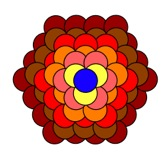This can then be explored in a similar way with older pupils but the layers are not quite so straightforward, as can be seen in these two pictures, that show the front balls on one "side" and the front balls on the adjacent "side".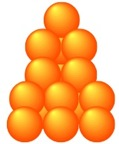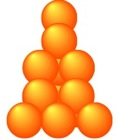So for this new irregular hexagonal based pyramid we'd have layers of 1, 3, 7, 12, 19, 27, 37, 48 ...

Each of these three sequences can be explored for different types of patterns. According to the pupils' experiences and skills, they may be asked to extend those sequences themselves or just be presented with them as shown.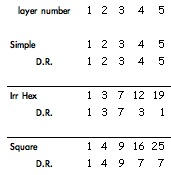(see 1 in the Notes )

You'll notice that I've just looked at the digital root (D.R. - see Digital Roots ) as this usually throws up some very interesting patterns, as it does in every example in this article.

It's good to bring in other aspects of mathematics to a challenge, so, as well as having so much numerical work here we can explore the shape and space aspects of it too! Some pupils would definitely prefer the visual - either creating their own or being presented with them. In that case, using layer 5 as a starting point we would have: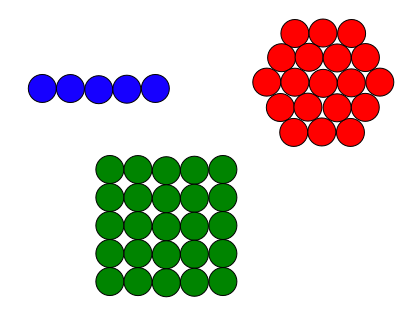Younger pupils may particularly like to explore the shape and space of the activity and ignore the numerical aspects.
-------------------------

Having got three lots of results, we can now pursue more mathematical processes.
For example we could look at the numerical differences of the numbers in each layer of the three arrangements.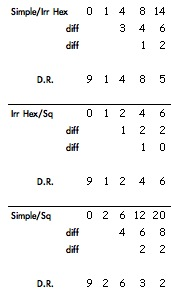(see 1 in the Notes )

Taking the differences as a sequence and looking at both their differences and the digital roots of them gives us more sequences to pursue.

Some pupils would benefit by looking at these differences as they would appear spatially.

This would be layer 5 for the three different arrangements. Taking a couple of the arrangements, e.g. simple and square, at a time, the differences can be seen and talked about in a variety of ways. Some pupils could look at what happens as more layers are added. So, looking at both layers 5 and 6 we could see (in the same order):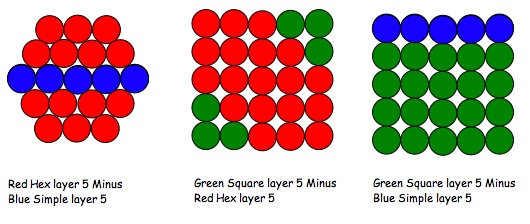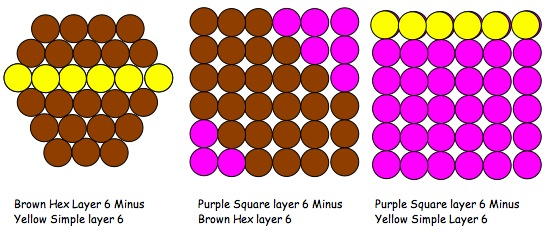The irregular hexagonal one has had to be skewed slightly to fit on top of the square.

The differences between the simple and square arrangements, as seen in the table and in the pictures above, could possibly show the presence of factors to many pupils - 4, 5 and 5, 6. So we could look at factors. Here I present two tables of the factors of those differences for pupils to explore and ask WHY? at various points, or for them to create themselves. (See also table 5b in the Notes .)
Firstly the differences between simple and irregular hexagonal, and the simple and square.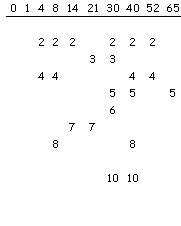(2a in Notes )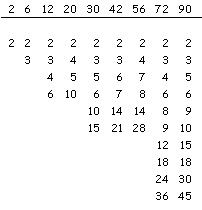(2b in Notes )

Again things that are noticed may be looked at visually, e.g. the occurrence of consecutive numbers in the factors of 2b. So for 2, 6, 12, 20 in the sequence we have factors - 2, 3, 4, 5.
Looking at it spacially, the simple (BLUE) overlapping the square (GREEN) so that the remaining Greens show the difference. It can then be seen - as below - why we get the numbers 2, 3, 4 and 5 as the factors. [As the differences in Green are 2x1, 3x2, 4x3, 5x4.]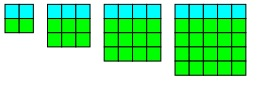-------------------------

It's always good to ask the question, "I wonder what would happen if I ...?" and to encourage pupils to do the same. So what would happen if we look at a set of stacks getting bigger each time, and explored them in a similar way? [I've called these, "totals".]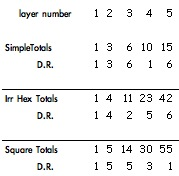and comparing the differences between different kinds of stacks: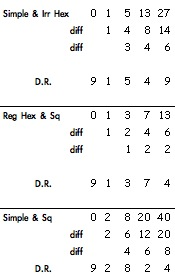(see 3 in the Notes )
-------------------------

We've looked at these three stacking arrangements and the sequences they've produced. The arrangements were related firstly to triangular, then square numbers. The newly introduced hexagonal one has a base which alternates between being regular and irregular.
To have hexagonal numbers in keeping with the triangular and square numbers we would have to miss out every other layer. This idea may very well come from some of the more experienced pupils.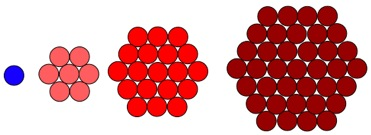So the exploration can continue by saying that we've looked at stacking and got involved with some triangular numbers [totals of "simple"] and square numbers, so what about ignoring the stacks and looking at triangular, square and hexagonal numbers? In other words, asking, "I wonder what would happen if I explore the three more common number patterns in the same way as the stacking exploration?"

These three patterns start off as: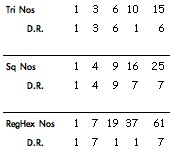(see 4 in Notes )

So, as before, having found it useful in producing patterns and ideas we look at the differences between these three newly generated number patters and the digital roots.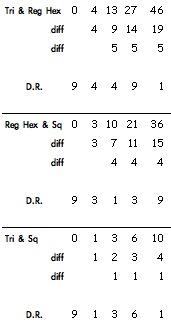(see 3 in Notes )

Again as we have three new sequences we might look at differences within each.
A reminder that the results that we got in this last table might be looked at visually.
Sometimes the shape has to be skewed slightly to show the subtraction [as shown below in the 2nd and 3rd ones]:

1.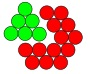2.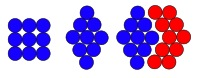3.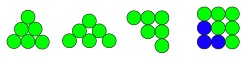So (1) shows tri number green that could overlap the red hex, so the remaining reds show the difference.
In (2) the square is skewed and then the diagram shows how it could overlap the red hex, so the remaining red show the difference.
(3) shows the tri number that is skewed and turned and then how it could overlap the blue square, so the remaining blues show the difference.

How these progress with the 4th, 5th, 6th etc. can be quite challenging in aspects of both number and shape and space.

These three number patterns can, of course, be looked at cumulatively. For example in the case of triangle numbers it would be:
1, 1+3, 1+3+6, 1+3+6+10 etc.

Do the same for all three number patterns and explore!
Then look at differences as we have done in the past!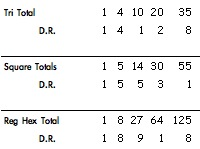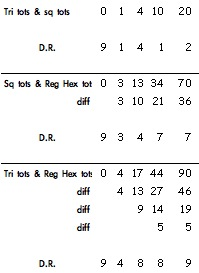(see 5 in Notes )
-------------------------

Just to whet your appetite, look at the numbers showing regular hexagonal totals i.e.
1, 1+7, 1+7+19, 1+7+19+37
Then consider cube numbers!!
Why?
Spatially?

-------------------------

Talking of Spatially - the younger/less experienced pupils can look at the spatial patterns in each of the hexagonal numbers, perhaps something like this: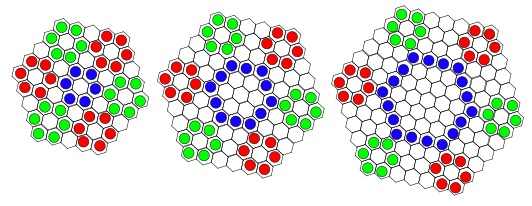or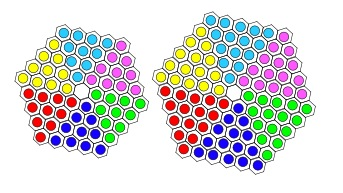-------------------------

Returning to the numbers ... Having got tables that show patterns in the three number sequences and patterns in their totals, we could compare things between the two and see if there is anything of interest - and if there is, WHY?. As an example, take the triangular numbers and totals of regular hexagon numbers and square numbers: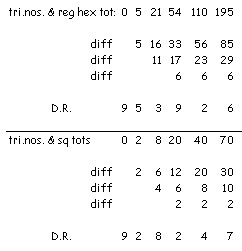(see 6 in Notes )

What about the presence of prime factors?

Well we could look at something in this table 6, like the difference between the triangular numbers and square number totals: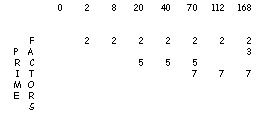(see 7 in Notes )

Also having got regular hexagon numbers we could look at the factors there: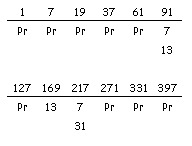(see 8 in Notes )

Quite a lot here with so many primes and the numbers that make up the prime factors!
An extension of this can be seen in the Notes showing 54 hexagonal numbers - with 27 primes!! - for exploring.
-------------------------

Those of us in England may wish to look at the Primary Framework , and there, in Using & Applying, say for Year 6, we would find the following.
All these statements could be experienced, used and learnt from the activities described above.

Using & Applying Mathematics Year 6

Solve multi-step problems, and problems;
Choose and use appropriate calculation strategies at each stage, including calculator use;
Tabulate systematically the information in a problem;
Identify and record the steps or calculations needed to solve it, using symbols where appropriate; interpret solutions in the original context and check their accuracy;
Suggest, plan and develop lines of enquiry; collect, organise and represent information, interpret results and review methods;
Represent and interpret sequences, patterns and relationships involving numbers and shapes; suggest and test hypotheses;
Construct and use simple expressions and formulae in words then symbols;
Explain reasoning and conclusions, using words, symbols or diagrams as appropriate.
-------------------------

I've tried to show an example of taking a fairly simple activity and getting questions going that lead to other explorations. To summarise:

Simple challenge;
Ask "I wonder what would happen if we did them differently?";
Look at any number patterns generated, explore digital roots;
Take a look at it all spatially if appropriate;
Compare one number pattern with another; also spatially;
Have a look at factors;
Ask "I wonder what would happen if we did cumulative totals?";
See if there are any divergent thoughts that come out from it so far that lead to further study;
[That could lead to starting all over again as I did by introducing the regular number sequences.]

So allow freedom, give ownership, praise all kinds of suggestions, and take risks.
It's all a bit like writing a poem.